### 通用别克 威朗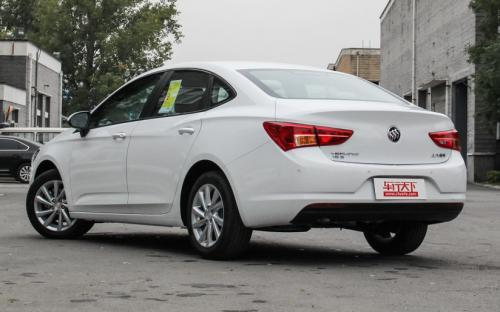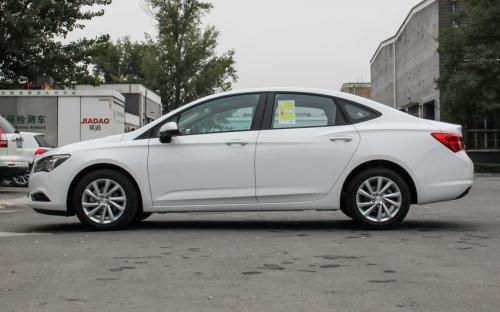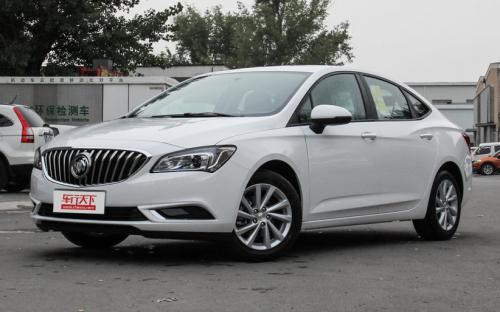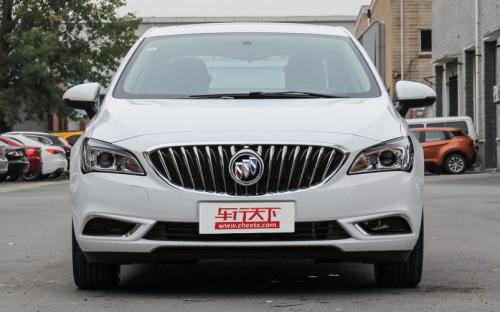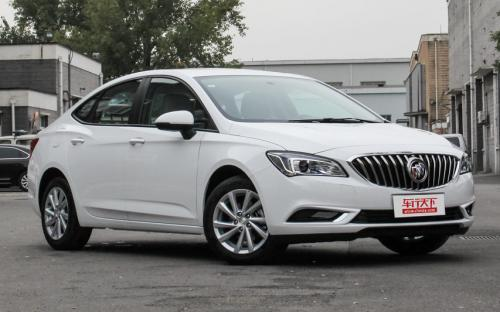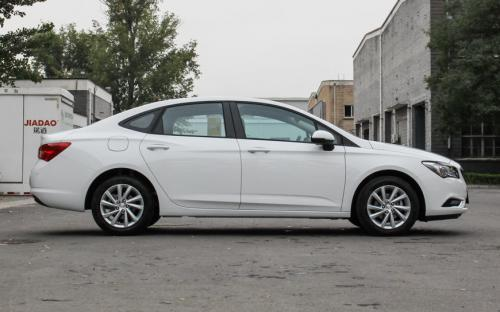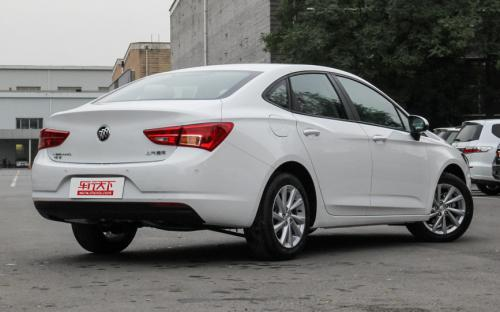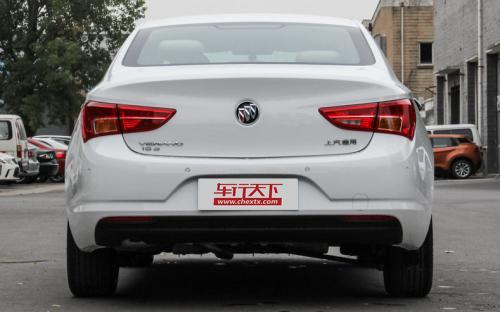0 种颜色可选2017款最低售价：13.59 万元起

4718(mm)1802(mm)1466(mm)##### 配置亮点：
• 胎压监测装置

• ISOFIX儿童座椅接口

• 车身稳定控制(ESC/ESP/DSC等)

• 电动天窗

• 定速巡航

• 后倒车雷达

• 真皮座椅

• GPS导航系统

• 氙气大灯

• 后视镜加热

• 提交
2017款 三厢 15S 自动领先型 (206张)
• 2017款 三厢 15S 自动领先型 (206张)
• 2017款 三厢 20T 双离合领先型 (125张)
• 2017款 三厢 15S 手动领先型 (133张)
• 通用别克 威朗 绕车实拍• 通用别克 威朗 在售车型

排量 车型 厂商指导价 本地最低报价 购车工具
1.5L
13.59万
13.59万

三厢 15S 自动进取型 6挡手自一体
14.69万
14.69万

14.49万
三厢 15S 自动领先型 6挡手自一体
15.49万
1.5T
16.29万
17.29万
19.99万
19.99万

通用别克 威朗 经销商

查看更多 >>

### 通用别克 威朗 动力加速

威朗 0-100公里加速时间分布在 8.8-13.2秒 属于 运动级

动力级别 加速时间 车型
民用级(2款)
12.5s
三厢 15S 手动进取型三厢 15S 手动领先型轿跑 15S 自动进取型GS 20T 双离合燃情运动型15S 手动进取型15S 手动领先型
13.2s
三厢 15S 自动进取型三厢 15S 自动领先型轿跑 15S 自动领先型GS 20T 双离合纵情运动型15S 自动进取型15S 自动领先型
运动级(2款)
8.8s
三厢 20T 双离合领先型三厢 20T 双离合精英型20T 双离合领先型20T 双离合精英型20T 双离合豪华型
8.9s
三厢 20T 双离合旗舰型20T 双离合旗舰型

通用别克 威朗 视频

通用别克 威朗 新闻资讯

# 别克GL6/阅朗等五款车型于10月16日上市

国产新车 超过10673次关注

日前，我们从官方获悉，上汽通用别克GL6、阅朗以及新款英朗、威朗、威朗GS这五款车型一同将于10月16日正式上市销售。

# 售价13.59-19.99万 别克新款威朗正式上市

上市新车 超过9395次关注

日前，上汽通用正式宣布，旗下2017款别克威朗正式上市，新车将推出两种动力共计7款车型，售价区间保持不变仍为13.59-19.99万元

# 外貌实力两手抓 试驾2016款别克威朗轿跑

试驾评测 超过9802次关注

现如今，汽车厂商对自己的家族式设计越发明显，在别克阵营中，威朗两厢版（威朗轿跑）就是在保留家族基因的同时又做到出其不意。今天试驾的1.5S自动领先型售价为15....

# 威朗轿跑/GS正式上市 售价14.59-20.59万

国产新车 超过8891次关注

2015年11月18日，上汽通用别克在广州车展前夕正式推出全新威朗轿跑/威朗GS并公布了他们的售价，新车根据不同配置共有5款车型，售价为14.59-20.59万元。

# 酷爱运动风 全新威朗GS到店实拍

国产新车 超过7879次关注

说起运动风跑车，你能想起来什么？是外表优雅的奥迪RS5，又或者是彰显个性的捷豹F-type？都不是，今天小编要给大家带来的这款车就是上海通用别克旗下的运动型轿跑威朗GS。

# 运动打造/配Carplay 威朗GS内饰官图

即将上市 超过6082次关注

我们从别克官方得到了别克威朗GS的内饰官图，作为通用别克旗下的一款紧凑车型，新车将会在11月的广州车展首发，并将于12月正式上市。

猜你喜欢

﻿
• 快速找车
• 选择品牌
• 选择品牌
• A  奥迪
• A  阿斯顿·马丁
• A  阿尔法·罗密欧
• B  宝沃
• B  布加迪
• B  巴博斯
• B  保时捷
• B  宾利
• B  奔驰
• B  宝马
• B  本田
• B  别克
• B  标致
• B  比亚迪
• B  宝骏
• B  北汽制造
• B  北汽新能源
• B  北汽幻速
• B  北汽威旺
• B  北京汽车
• B  奔腾
• B  北汽绅宝
• C  长安
• C  长安商用
• C  长城
• C  昌河
• D  大众
• D  道奇
• D  DS
• D  东南
• D  东风风神
• D  东风风行
• D  东风小康
• D  东风风度
• D  东风
• F  福特
• F  丰田
• F  菲亚特
• F  法拉利
• F  福田
• F  福迪
• F  福汽启腾
• G  观致
• G  广汽传祺
• G  广汽吉奥
• G  GMC
• H  红旗
• H  汉腾汽车
• H  哈弗
• H  哈飞
• H  海格
• H  海马
• H  华颂
• H  黄海
• H  华泰
• H  恒天
• J  吉利汽车
• J  捷豹
• J  Jeep
• J  江淮
• J  江铃
• J  金杯
• J  九龙
• J  金旅
• K  凯翼
• K  凯迪拉克
• K  克莱斯勒
• K  科尼塞克
• K  卡威
• K  开瑞
• L  路虎
• L  林肯
• L  劳斯莱斯
• L  兰博基尼
• L  雷克萨斯
• L  铃木
• L  雷诺
• L  理念
• L  力帆
• L  莲花汽车
• L  猎豹
• L  路特斯
• L  陆风
• M  马自达
• M  MG
• M  MINI
• M  玛莎拉蒂
• M  摩根
• M  迈凯轮
• N  纳智捷
• O  欧宝
• O  讴歌
• O  欧朗
• Q  奇瑞
• Q  起亚
• Q  启辰
• R  日产
• R  荣威
• R  瑞麒
• S  三菱
• S  斯威汽车
• S  萨博
• S  smart
• S  斯柯达
• S  斯巴鲁
• S  思铭
• S  双龙
• S  上汽大通
• S  双环
• T  特斯拉
• T  腾势
• W  沃尔沃
• W  五菱汽车
• W  五十铃
• W  威兹曼
• W  威麟
• X  现代
• X  雪佛兰
• X  雪铁龙
• X  西雅特
• Y  一汽
• Y  英菲尼迪
• Y  英致
• Y  依维柯
• Y  野马汽车
• Y  永源
• Z  众泰
• Z  中华
• Z  中兴
• Z  知豆
• 选择车系
• 选择车系
• 车型对比
• 选择品牌
• 选择品牌
• A  奥迪
• A  阿斯顿·马丁
• A  阿尔法·罗密欧
• B  宝沃
• B  布加迪
• B  巴博斯
• B  保时捷
• B  宾利
• B  奔驰
• B  宝马
• B  本田
• B  别克
• B  标致
• B  比亚迪
• B  宝骏
• B  北汽制造
• B  北汽新能源
• B  北汽幻速
• B  北汽威旺
• B  北京汽车
• B  奔腾
• B  北汽绅宝
• C  长安
• C  长安商用
• C  长城
• C  昌河
• D  大众
• D  道奇
• D  DS
• D  东南
• D  东风风神
• D  东风风行
• D  东风小康
• D  东风风度
• D  东风
• F  福特
• F  丰田
• F  菲亚特
• F  法拉利
• F  福田
• F  福迪
• F  福汽启腾
• G  观致
• G  广汽传祺
• G  广汽吉奥
• G  GMC
• H  红旗
• H  汉腾汽车
• H  哈弗
• H  哈飞
• H  海格
• H  海马
• H  华颂
• H  黄海
• H  华泰
• H  恒天
• J  吉利汽车
• J  捷豹
• J  Jeep
• J  江淮
• J  江铃
• J  金杯
• J  九龙
• J  金旅
• K  凯翼
• K  凯迪拉克
• K  克莱斯勒
• K  科尼塞克
• K  卡威
• K  开瑞
• L  路虎
• L  林肯
• L  劳斯莱斯
• L  兰博基尼
• L  雷克萨斯
• L  铃木
• L  雷诺
• L  理念
• L  力帆
• L  莲花汽车
• L  猎豹
• L  路特斯
• L  陆风
• M  马自达
• M  MG
• M  MINI
• M  玛莎拉蒂
• M  摩根
• M  迈凯轮
• N  纳智捷
• O  欧宝
• O  讴歌
• O  欧朗
• Q  奇瑞
• Q  起亚
• Q  启辰
• R  日产
• R  荣威
• R  瑞麒
• S  三菱
• S  斯威汽车
• S  萨博
• S  smart
• S  斯柯达
• S  斯巴鲁
• S  思铭
• S  双龙
• S  上汽大通
• S  双环
• T  特斯拉
• T  腾势
• W  沃尔沃
• W  五菱汽车
• W  五十铃
• W  威兹曼
• W  威麟
• X  现代
• X  雪佛兰
• X  雪铁龙
• X  西雅特
• Y  一汽
• Y  英菲尼迪
• Y  英致
• Y  依维柯
• Y  野马汽车
• Y  永源
• Z  众泰
• Z  中华
• Z  中兴
• Z  知豆
• 选择车系
• 选择车系
• 选择车型
• 选择车型
• 意见反馈